# Introduction to Bayesian Optimization

## Goal¶

This notebook aims to introduce how Bayesian Optimization works using bayesian-optimization module.

Bayesian Optimization is the way of estimating the unknown function where we can choose the arbitrary input $x$ and obtain the response from that function. The outcome of Bayesian Optimization is to obtain the mean and confidence interval of the function we look for by step. You could also stop earlier or decide go further iteratively.

This will cover the very first toy example of Bayesian Optimization by defining "black-box" function and show how interactively or step-by-step Bayesian Optimization will figure and estimate this "black-box" function.

Reference

## Libraries¶

In :
from bayes_opt import BayesianOptimization
from bayes_opt import UtilityFunction
import numpy as np
import warnings
from IPython.display import display, clear_output
import matplotlib.pyplot as plt
from matplotlib import gridspec
%matplotlib inline


## Unknown Function¶

We can have any function to estimate here. As an example, we will have 1-D function defined by the following equation:

$$f(x) = 3e^{-(x-3)^{2}} - e^{-(x-2)^2} + 2 e^{-(x+3)^2}$$
In :
def unknown_func(x):
return 3 * np.exp(-(x-3) **2) - np.exp(-(x-2) **2) + 2 * np.exp(-(x + 3) **2)


If we visualize the unknown function (as a reference), we can plot like below. Note that we are not supposed to know this plot since this function is "black-box"

In :
x = np.linspace(-6, 6, 10000).reshape(-1, 1)
y = unknown_func(x)

plt.plot(x, y);
plt.title('1-D Unknown Function to be estimated');
plt.xlabel('X');
plt.ylabel('Response from the function');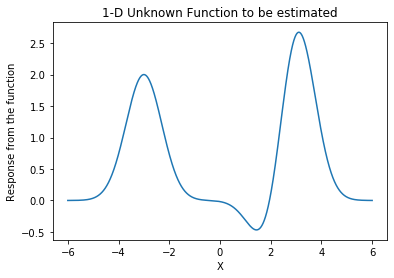## Bayesian Optimization¶

First of all, we need to create BayesianOptimization object by passing the function f you want to estimate with its input boundary as pbounds.

In :
optimizer = BayesianOptimization(f=unknown_func, pbounds={'x': (-6, 6)}, verbose=0)
optimizer

Out:
<bayes_opt.bayesian_optimization.BayesianOptimization at 0x11ab55dd8>

Then, we can start to explore this function by trying different inputs.

• init_points is the number of initial points to start with.
• n_iter is the number of iteration. This optimizer.maximize hold the state so whenever you execute it, it will continue from the last iteration.

### Helper functions¶

In :
def posterior(optimizer, x_obs, y_obs, grid):
optimizer._gp.fit(x_obs, y_obs)

mu, sigma = optimizer._gp.predict(grid, return_std=True)
return mu, sigma

def plot_gp(optimizer, x, y, fig=None, xlim=None):
if fig is None:
fig = plt.figure(figsize=(16, 10))
steps = len(optimizer.space)
fig.suptitle(
'Gaussian Process and Utility Function After {} Steps'.format(steps),
fontdict={'size':30}
)

gs = gridspec.GridSpec(2, 1, height_ratios=[3, 1])
axis = plt.subplot(gs)
acq = plt.subplot(gs)

x_obs = np.array([[res["params"]["x"]] for res in optimizer.res])
y_obs = np.array([res["target"] for res in optimizer.res])

mu, sigma = posterior(optimizer, x_obs, y_obs, x)
axis.plot(x, y, linewidth=3, label='Target')
axis.plot(x_obs.flatten(), y_obs, 'D', markersize=8, label=u'Observations', color='r')
axis.plot(x, mu, '--', color='k', label='Prediction')

axis.fill(np.concatenate([x, x[::-1]]),
np.concatenate([mu - 1.9600 * sigma, (mu + 1.9600 * sigma)[::-1]]),
alpha=.3, fc='C0', ec='None', label='95% confidence interval')
if xlim is not None:
axis.set_xlim(xlim)
axis.set_ylim((None, None))
axis.set_ylabel('f(x)', fontdict={'size':20})
axis.set_xlabel('x', fontdict={'size':20})

utility_function = UtilityFunction(kind="ucb", kappa=5, xi=0)
utility = utility_function.utility(x, optimizer._gp, 0)
acq.plot(x, utility, label='Utility Function', color='C3')
acq.plot(x[np.argmax(utility)], np.max(utility), 'o', markersize=15,
label=u'Next Best Guess', markerfacecolor='gold', markeredgecolor='k', markeredgewidth=1)
if xlim is not None:
acq.set_xlim(xlim)
acq.set_ylim((np.min(utility) , np.max(utility) + 0.5))
acq.set_ylabel('Utility', fontdict={'size':20})
acq.set_xlabel('x', fontdict={'size':20})

return fig


### Visualize the iterative step¶

In :
# fig = plt.figure(figsize=(16, 10))
xlim = (-6, 6)
optimizer = BayesianOptimization(f=unknown_func, pbounds={'x': xlim}, verbose=0)

with warnings.catch_warnings():
warnings.simplefilter("ignore")
for i in range(15):
break
#         optimizer.maximize(init_points=0, n_iter=1, kappa=5)
#         fig = plot_gp(optimizer, x, y, fig=fig, xlim=xlim)
#         display(plt.gcf())
#         clear_output(wait=True)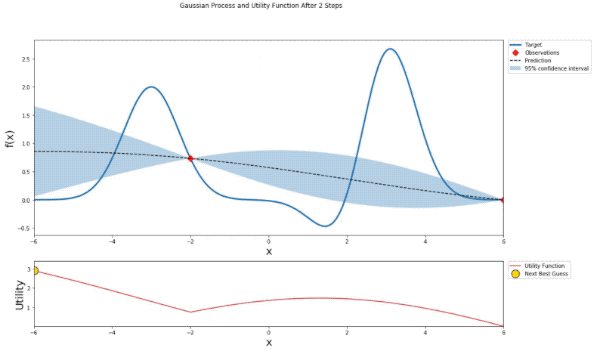# cProfile Examples in Python

## Goal¶

This post aims to introduce how to use cProfile to measure the running time for each statement and find the bottleneck of your program.

## Libraries¶

In :
import cProfile


In :
def linear_func(n):
for i in range(n):
pass
return

for i in range(n):
for i in range(n):
pass

return

def exp_func(n):
if n <= 1:
return n
return exp_func(n-1) + exp_func(n-2)

In :
def main_func(n):
linear_func(n)
exp_func(n)


## Profile the main function¶

In :
cProfile.run('main_func(n=20)')

         21897 function calls (7 primitive calls) in 0.006 seconds

Ordered by: standard name

ncalls  tottime  percall  cumtime  percall filename:lineno(function)
1    0.000    0.000    0.000    0.000 <ipython-input-37-393400eb079b>:1(linear_func)
21891/1    0.006    0.000    0.006    0.006 <ipython-input-37-393400eb079b>:13(exp_func)
1    0.000    0.000    0.000    0.000 <ipython-input-37-393400eb079b>:6(quad_func)
1    0.000    0.000    0.006    0.006 <ipython-input-38-1333493d3326>:1(main_func)
1    0.000    0.000    0.006    0.006 <string>:1(<module>)
1    0.000    0.000    0.006    0.006 {built-in method builtins.exec}
1    0.000    0.000    0.000    0.000 {method 'disable' of '_lsprof.Profiler' objects}



## How to read the result¶

• ncalls is the number of calls.
• tottime is a total of the time spent.
• percall is the average time for each call, i.e., tottime divided by ncalls
• cumtime is the cumulative time spent.
• (2nd) percall is the quotient of cumtime divided by primitive calls
• filename:lineno(function) indicates the information about the function with the format "{file name}:{line number}{function name}"

# Time Series Forecasting for Daily Births Dataset by Prophet

## Goal¶

This post aims to introduce how to forecast the time series data for daily births dataset using Prophet.

Prohpeht Forecasting Model

$$y (y) = g(t) + s(t) + h(t) + \epsilon_t$$
• $g(t)$: a growth term, which is a trend curve
• $s(t)$: a seasonality term, which periodically changes
• $h(t)$: a holiday term, which indicates irregular events (given by users)
• $\epsilon_t$: an error term

Reference

## Libraries¶

In :
import pandas as pd
import numpy as np
import fbprophet
import warnings
import matplotlib.pyplot as plt
%matplotlib inline


## Load a female daily births dataset¶

In :
url = "https://raw.githubusercontent.com/jbrownlee/Datasets/master/daily-total-female-births.csv"
df.columns = ['ds', 'y']

Out:
ds y
0 1959-01-01 35
1 1959-01-02 32
2 1959-01-03 30
3 1959-01-04 31
4 1959-01-05 44

### Visualize the raw data¶

In :
plt.plot(df['ds'], df['y']);
plt.title('Daily Female Births in 1959');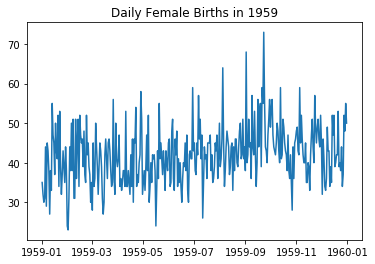## Create a Prophet instance¶

In :
with warnings.catch_warnings():
warnings.simplefilter("ignore")
m = fbprophet.Prophet(yearly_seasonality=True, daily_seasonality=False,
changepoint_range=0.9,
changepoint_prior_scale=0.5,
seasonality_mode='multiplicative')
m.fit(df);

In :
future = m.make_future_dataframe(periods=50, freq='d')
forecast = m.predict(future)


## Visualize¶

### By Component¶

In :
m.plot_components(forecast);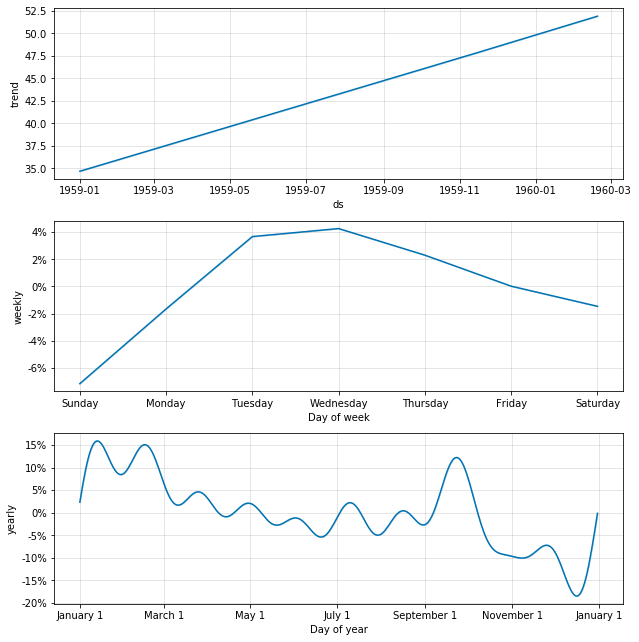### Plot raw data with forecast¶

In :
m.plot(forecast);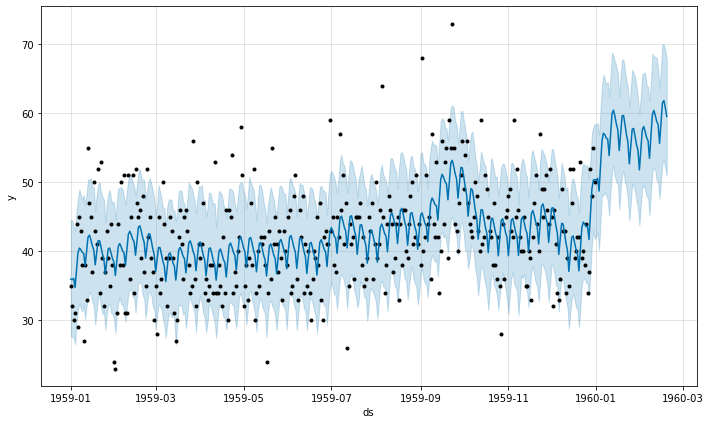## Change point detection¶

No change is detected.

In :
fig = m.plot(forecast)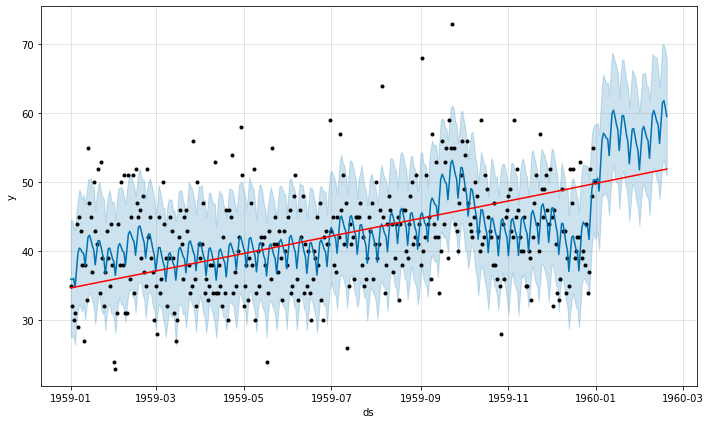# Prophet 101: a time-series forecasting module

## Goal¶

This post aims to introduce the basics of Prophet, which is a time-series forecasting module implemented by Facebook.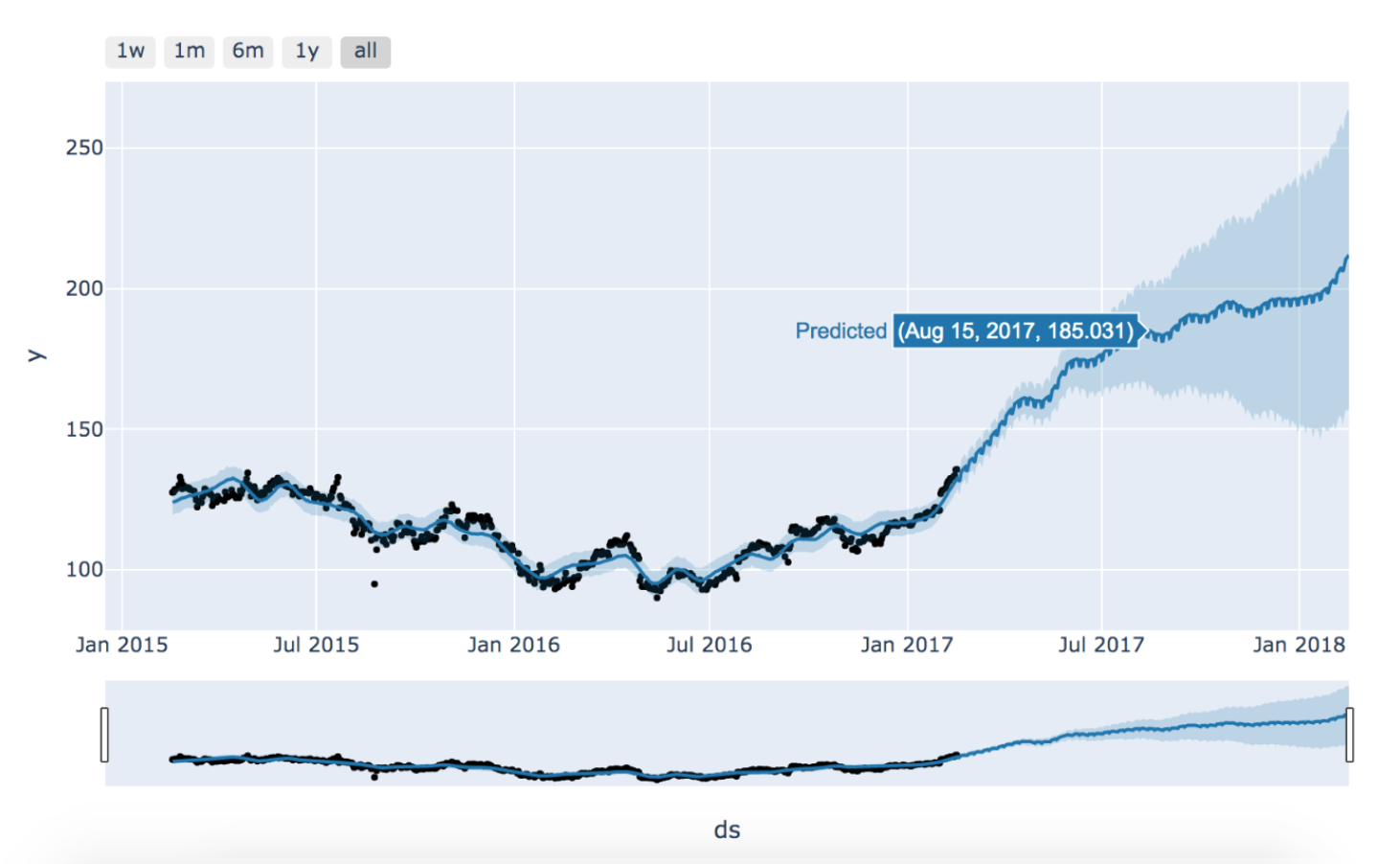Reference

# Gradient descent implementation from scratch

## Goal¶

This post aims to introduce how to implement Gradient Descent from scratch.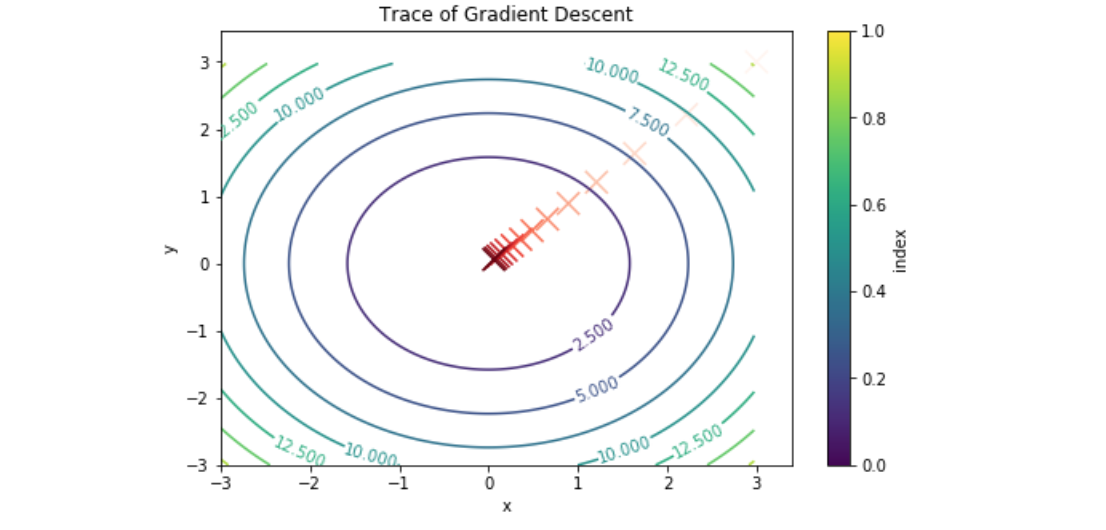Reference

## Libraries¶

In [ ]:
import pandas as pd
import numpy as np
import matplotlib.pyplot as plt
%matplotlib inline


## Define the function to be explored¶

In :
def surface_function(x):
return np.sum(x**2)

In :
delta = 0.025
x = np.arange(-3.0, 3.0, delta)
y = np.arange(-3.0, 3.0, delta)
X, Y = np.meshgrid(x, y)
fig, ax = plt.subplots()
CS = ax.contour(X, Y, Z)
ax.clabel(CS, inline=1, fontsize=10);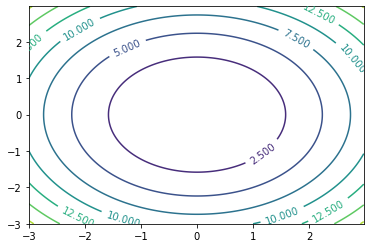## Compute gradient given a function¶

In :
def numerical_gradient(f, x0):
h = 1e-5

for i in range(x0.size):
tmp = x0[i]

# compute f(x0 + h)
x0[i] = tmp + h
fx0_p = f(x0)

# compute f(x0 - h)
x0[i] = tmp - h
fx0_m = f(x0)

grad[i] = (fx0_p - fx0_m) / (2*h)
x0[i] = tmp


In :
numerical_gradient(surface_function, np.array([0., 1.]))

Out:
array([0., 2.])

In :
class GradientDescent:
def __init__(self):
self.trace = []

def gradient_descent(self, f, init_x, lr=0.01, steps=200):
x = init_x
for i in range(steps):
self.trace.append(list(x))

return x

In :
init = np.array([3., 3.])

Out:
array([0.05276384, 0.05276384])

## Plot the trace on the surface function¶

In :
df_trace = pd.DataFrame(gd.trace, columns=['x', 'y']).reset_index()

Out:
index x y
0 0 3.000000 3.000000
1 1 2.940000 2.940000
2 2 2.881200 2.881200
3 3 2.823576 2.823576
4 4 2.767104 2.767104
In :
fig, ax = plt.subplots(figsize=(8, 5))
CS = ax.contour(X, Y, Z)
ax.clabel(CS, inline=1, fontsize=10);
df_trace.iloc[::15, :].plot(kind='scatter', x='x', y='y', c='index', cmap='Reds', marker='x', s=200,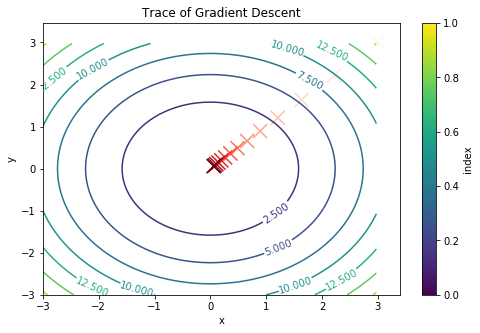# Saving Machine Learning Models by joblib

## Goal¶

This post aims to introduce how to save the machine learning model using joblib.

## Libraries¶

In :
import pandas as pd
import numpy as np
from sklearn.linear_model import LinearRegression
import joblib


## Create a data¶

In :
boston = load_boston()
X, y = boston.data, boston.target


## Train a model¶

In :
reg = LinearRegression()
reg.fit(X, y)

Out:
LinearRegression(copy_X=True, fit_intercept=True, n_jobs=None,
normalize=False)

## Save the model as pickle¶

In :
joblib.dump(reg, 'linear_regression.pkl')

Out:
['linear_regression.pkl']

### check the saved model¶

In :
!ls | grep linear*pkl

linear_regression.pkl


In :
loaded_reg = joblib.load('linear_regression.pkl')

Out:
LinearRegression(copy_X=True, fit_intercept=True, n_jobs=None,
normalize=False)

# Explain the interaction values by SHAP

## Goal¶

This post aims to introduce how to explain the interaction values for the model's prediction by SHAP. In this post, we will use data NHANES I (1971-1974) from National Health and Nutrition Examaination Survey.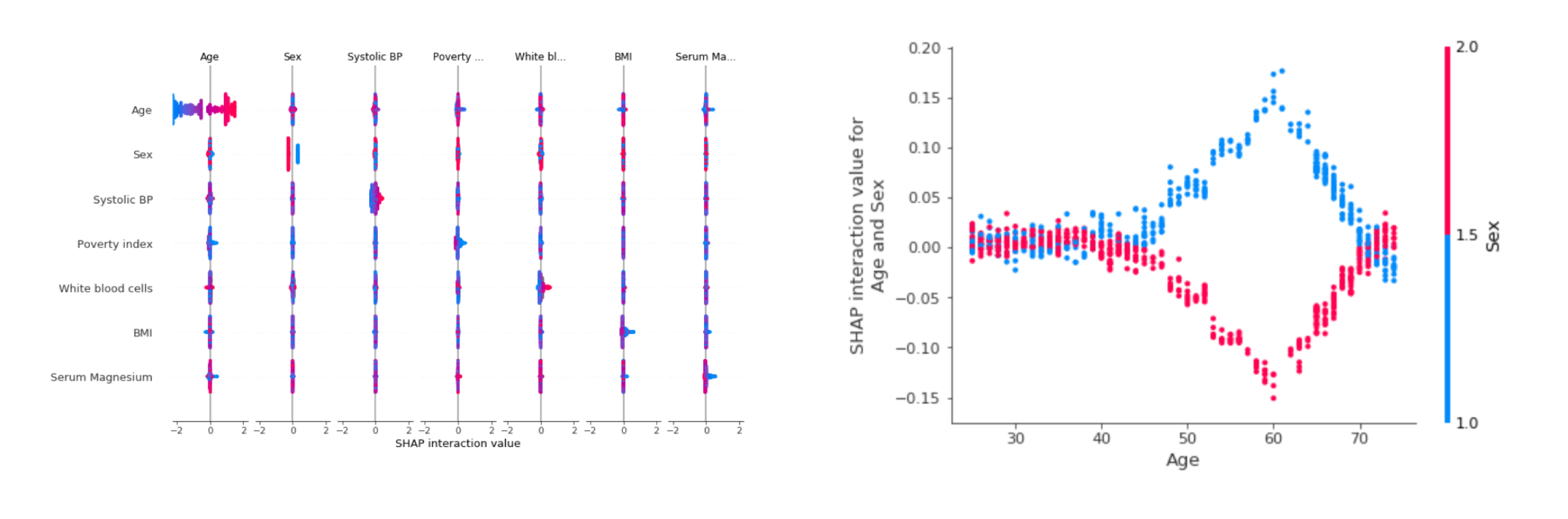Reference

# Explain the prediction for ImageNet using SHAP

## Goal¶

This post aims to introduce how to explain the prediction for ImageNet using SHAP.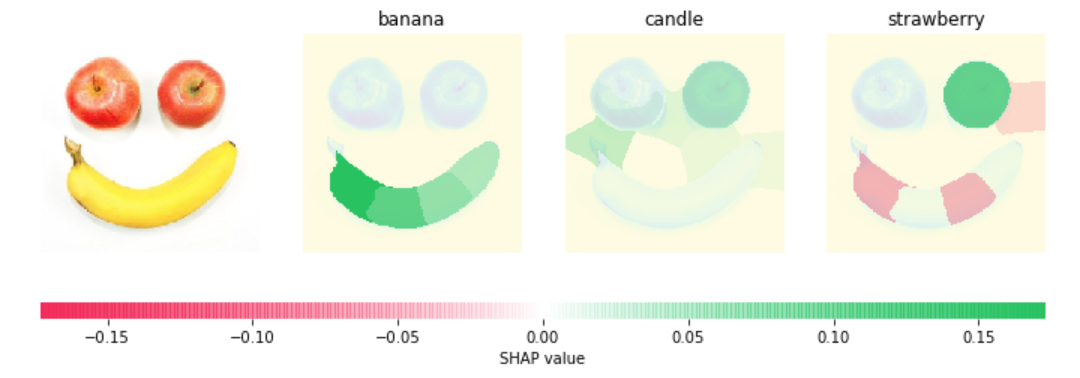Reference

## Libraries¶

In :
import keras
from keras.applications.vgg16 import VGG16, preprocess_input, decode_predictions
from keras.preprocessing import image
import requests
from skimage.segmentation import slic
import pandas as pd
import numpy as np
import matplotlib.pyplot as plt
import shap
import warnings

%matplotlib inline


## Configuration¶

In :
# make a color map
from matplotlib.colors import LinearSegmentedColormap
colors = []
for l in np.linspace(1, 0, 100):
colors.append((245 / 255, 39 / 255, 87 / 255, l))
for l in np.linspace(0, 1, 100):
colors.append((24 / 255, 196 / 255, 93 / 255, l))
cm = LinearSegmentedColormap.from_list("shap", colors)


In :
# load model data
r = requests.get('https://s3.amazonaws.com/deep-learning-models/image-models/imagenet_class_index.json')
feature_names = r.json()
model = VGG16()

WARNING:tensorflow:From /Users/hiro/anaconda3/envs/py367/lib/python3.6/site-packages/tensorflow/python/framework/op_def_library.py:263: colocate_with (from tensorflow.python.framework.ops) is deprecated and will be removed in a future version.
Instructions for updating:
Colocations handled automatically by placer.
553467904/553467096 [==============================] - 62s 0us/step


In :
# load an image
file = "../images/apple-banana.jpg"
img_orig = image.img_to_array(img)
plt.imshow(img);
plt.axis('off');### Segmentation¶

In :
# Create segmentation to explain by segment, not every pixel
segments_slic = slic(img, n_segments=30, compactness=30, sigma=3)

plt.imshow(segments_slic);
plt.axis('off');In :
segments_slic

Out:
array([[ 0,  0,  0, ...,  4,  4,  4],
[ 0,  0,  0, ...,  4,  4,  4],
[ 0,  0,  0, ...,  4,  4,  4],
...,
[22, 22, 22, ..., 21, 21, 21],
[22, 22, 22, ..., 21, 21, 21],
[22, 22, 22, ..., 21, 21, 21]])

## Utility Functions for masking and preprocessing¶

In :
# define a function that depends on a binary mask representing if an image region is hidden

if background is None:
background = image.mean((0, 1))

# Create an empty 4D array
out = np.zeros((zs.shape,
image.shape,
image.shape,
image.shape))

for i in range(zs.shape):
out[i, :, :, :] = image
for j in range(zs.shape):
if zs[i, j] == 0:
out[i][segmentation == j, :] = background
return out

def f(z):
return model.predict(

def fill_segmentation(values, segmentation):
out = np.zeros(segmentation.shape)
for i in range(len(values)):
out[segmentation == i] = values[i]
return out

In :
masked_images = mask_image(np.zeros((1,50)), segments_slic, img_orig, 255)

plt.axis('off');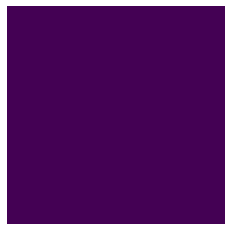## Create an explainer and shap values¶

In :
# use Kernel SHAP to explain the network's predictions
explainer = shap.KernelExplainer(f, np.zeros((1,50)))

with warnings.catch_warnings():
warnings.simplefilter("ignore")
shap_values = explainer.shap_values(np.ones((1,50)), nsamples=100) # runs VGG16 1000 times


## Obtain the prediction with the highest probability¶

In :
predictions = model.predict(preprocess_input(np.expand_dims(img_orig.copy(), axis=0)))
top_preds = np.argsort(-predictions)

In :
pd.Series(data={feature_names[str(inds[i])]:predictions[0, inds[i]]  for i in range(10)}).plot(kind='bar', title='Top 10 Predictions');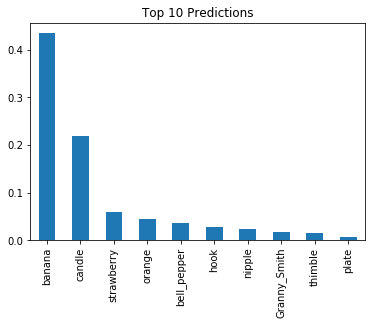## Explain the prediction by visualization¶

The following image is explained well for banana.

In :
# Visualize the explanations
fig, axes = plt.subplots(nrows=1, ncols=4, figsize=(12,4))
inds = top_preds
axes.imshow(img)
axes.axis('off')

max_val = np.max([np.max(np.abs(shap_values[i][:,:-1])) for i in range(len(shap_values))])
for i in range(3):
m = fill_segmentation(shap_values[inds[i]], segments_slic)
axes[i+1].set_title(feature_names[str(inds[i])])
axes[i+1].imshow(np.array(img.convert('LA'))[:, :, 0], alpha=0.15)
im = axes[i+1].imshow(m, cmap=cm, vmin=-max_val, vmax=max_val)
axes[i+1].axis('off')
cb = fig.colorbar(im, ax=axes.ravel().tolist(), label="SHAP value", orientation="horizontal", aspect=60)
cb.outline.set_visible(False)
plt.show()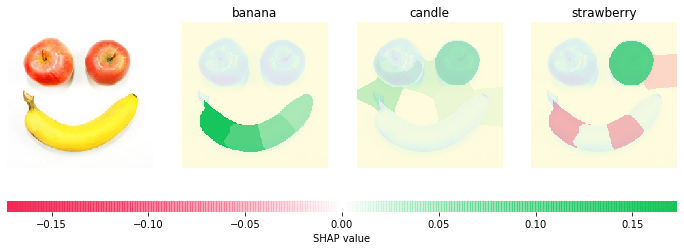# Sentiment Analysis by SHAP with Logistic Regression

## Goal¶

This post aims to introduce how to do sentiment analysis using SHAP with logistic regression.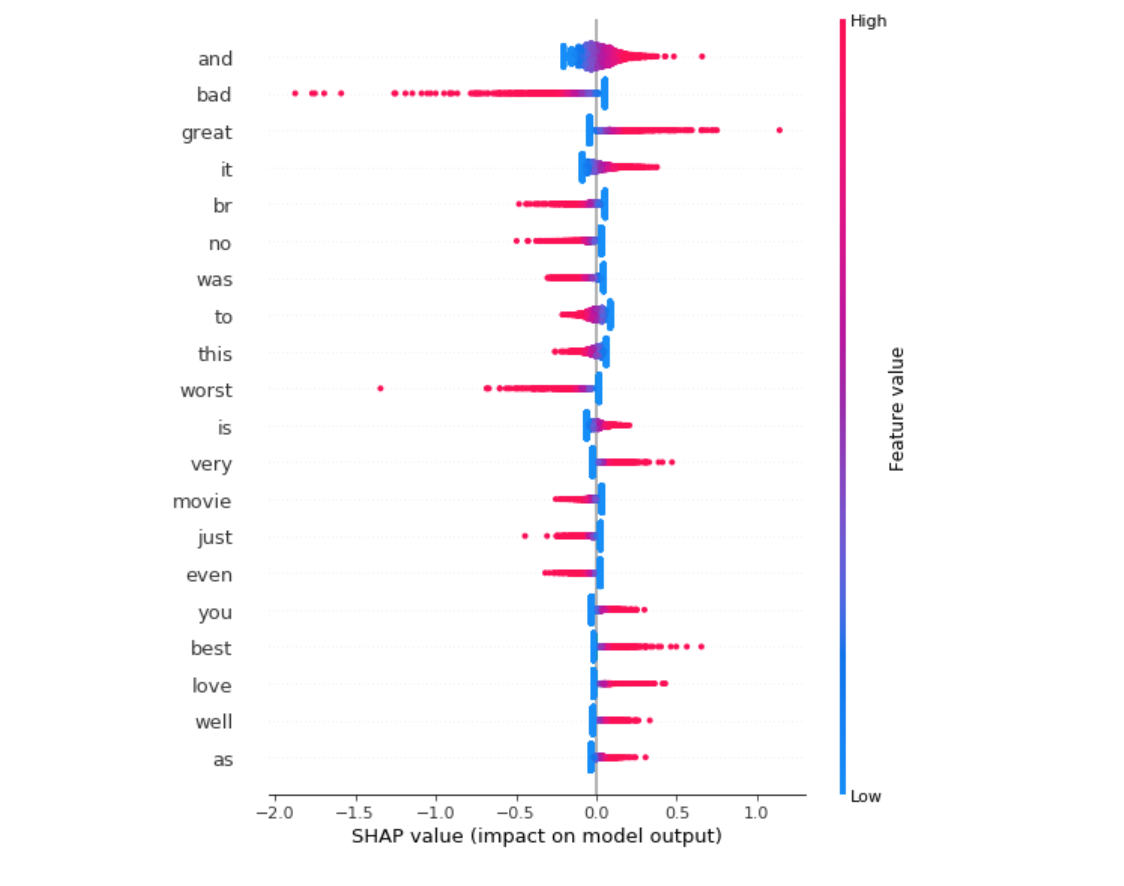Reference

# Explain Iris classification by SHAP

## Goal¶

This post aims to introduce how to explain Iris classification by SHAP.

Reference

## Libraries¶

In :
import sklearn
from sklearn.model_selection import train_test_split
import numpy as np
import shap
import time
shap.initjs()